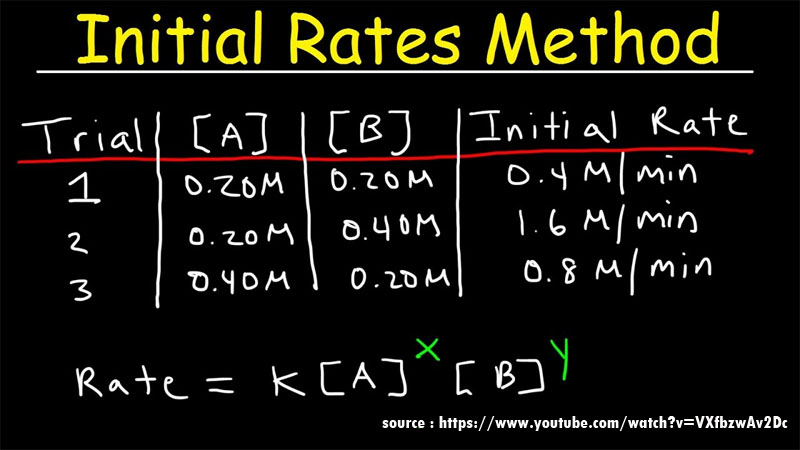### How To Know The Order Of The Reaction?

Order of the reaction is the part of the chemical reaction which determines the relationship between the rate of a chemical reaction and the concentration of the species involved in the chemical reaction. Order of the reaction depends on the chemical concentration of the reactants involved in the chemical reaction.

The first-order reaction dependents entirely on the concentration of one of the reactants involved in the chemical reaction. Some characteristics of the reaction in order of reaction are listed below.

• The number of species of reactants involved along with the concentration of the reactants directly affects the rate of reaction.
• The value of the order of reaction can be in the form of a fraction or an integer. It can even have a value of zero.
• The order of a reaction is not dependent on the stoichiometric coefficients that are corresponding to each species in the balanced chemical reaction.
• It can be obtained by adding all the exponents of the concentration terms in the rate expression.
• The reaction order of a chemical reaction is defined with the help of reactant concentrations and not with product concentrations.

The rate of the reaction is determined by rate law or rate equation and is given by

r = k[A]x[B]y

Where,

[A] and [B] = concentration of the species involved in a chemical reaction

x and y = partial orders of reaction for A and B

r = rate of reaction

k= rate constant

Overall reaction = x+y

### Know The Energy

How To Know The Order Of The Reaction? Read More